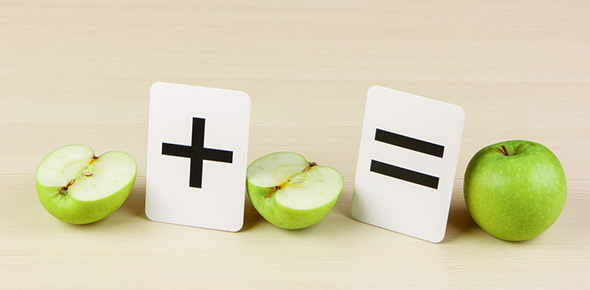# What Do You Know About Equivalent Fractions?

8 Questions | Total Attempts: 410SettingsThis quiz assesses your knowledge of fractions, mixed numbers and finding equivalents. So, let's try out this quiz. All the best!

• 1.
A fraction is a number that tells how a part of an object or a set compares with a whole.
• A.

True

• B.

False

• 2.
The sum of a whole number and a fraction is
• A.

Fraction

• B.

Equivalent fraction

• C.

Mixed number

• D.

Least common multiple

• 3.
The top number in a fraction that tells how many parts of a whole to consider is
• A.

Denominator

• B.

Numerator

• 4.
What operation do you use when solving equivalent fractions?
• A.

• B.

Subtracting

• C.

Multiplication

• D.

Division

• 5.
True or false: three sixths (3/6) is in lowest terms?
• A.

True

• B.

False

• 6.
True or false: 3/7 is equivalent to 12/28?
• A.

True

• B.

False

• 7.
When reducing a fraction, what operation do you use?
• A.

• B.

Subtracting

• C.

Multiplication

• D.

Division

• 8.
Fractions that name the same part of a whole are ___________________.
• A.

In lowest terms

• B.

Mixed numbers

• C.

Equivalent fractions

• D.

Numberator

Related TopicsBack to top